$$\newcommand{\xv}{\mathbf{x}} \newcommand{\Xv}{\mathbf{X}} \newcommand{\yv}{\mathbf{y}} \newcommand{\Yv}{\mathbf{Y}} \newcommand{\zv}{\mathbf{z}} \newcommand{\av}{\mathbf{a}} \newcommand{\Wv}{\mathbf{W}} \newcommand{\wv}{\mathbf{w}} \newcommand{\betav}{\mathbf{\beta}} \newcommand{\gv}{\mathbf{g}} \newcommand{\Hv}{\mathbf{H}} \newcommand{\dv}{\mathbf{d}} \newcommand{\Vv}{\mathbf{V}} \newcommand{\vv}{\mathbf{v}} \newcommand{\tv}{\mathbf{t}} \newcommand{\Tv}{\mathbf{T}} \newcommand{\Sv}{\mathbf{S}} \newcommand{\Gv}{\mathbf{G}} \newcommand{\zv}{\mathbf{z}} \newcommand{\Zv}{\mathbf{Z}} \newcommand{\Norm}{\mathcal{N}} \newcommand{\muv}{\boldsymbol{\mu}} \newcommand{\sigmav}{\boldsymbol{\sigma}} \newcommand{\phiv}{\boldsymbol{\phi}} \newcommand{\Phiv}{\boldsymbol{\Phi}} \newcommand{\Sigmav}{\boldsymbol{\Sigma}} \newcommand{\Lambdav}{\boldsymbol{\Lambda}} \newcommand{\half}{\frac{1}{2}} \newcommand{\argmax}{\underset{#1}{\operatorname{argmax}}} \newcommand{\argmin}{\underset{#1}{\operatorname{argmin}}} \newcommand{\dimensionbar}{\underset{#1}{\operatorname{|}}} \newcommand{\dimensionbar}{\underset{#1}{\operatorname{|}}} \newcommand{\grad}{\mathbf{\nabla}} \newcommand{\ebx}{e^{\betav_{#1}^T \xv_n}} \newcommand{\eby}{e^{y_{n,#1}}} \newcommand{\Tiv}{\mathbf{Ti}} \newcommand{\Fv}{\mathbf{F}} \newcommand{\ones}{\mathbf{1}_{#1}}$$

# Classification with Nonlinear Logistic Regression Using Neural Networks¶

12.1: Replaced note that mentioned objectiveF with note mentioning error_f.

## Motivation and Setup¶

Linear function approximator as a neural network.What must we add to do logistic regression?

Just add the softmax calculation to the output layer.Any thoughts on how to do nonlinear logistic regression?## Derivation¶

We will maximize the log likelihood of the training data. \begin{align*} L(\Wv) & = \left ( \prod_{n=1}^N \prod_{k=1}^K P(C=k|\xv_n)^{t_{n,k}} \right ) \\ \log L(\Wv) & = LL(\Wv) = \sum_{n=1}^N \sum_{k=1}^K t_{n,k} \log P(C=k|\xv_n)\\ & = \sum_{n=1}^N \sum_{k=1}^K t_{n,k} \log g_{n,k} \end{align*}

## Gradient of the Log Likelihood¶

\begin{align*} LL(\Wv) & = \sum_{n=1}^N \sum_{k=1}^K t_{n,k} \log g_{n,k}\;\;\;\;\; \text{ where } g_{n,k} = \frac{\eby{k}}{\sum_{m=1}^K \eby{m}}; \\ \frac{\partial LL(\Wv)}{\partial \Wv_{d,j}} & = \sum_{n=1}^N \sum_{k=1}^K \frac{t_{n,k}}{g_{n,k}} \frac{\partial g_{n,k}}{\partial \Wv_{d,j}}\\ \end{align*}

## Linear Version¶

\begin{align*} \frac{\partial LL(\Wv)}{\partial \Wv_{d,j}} & = \sum_{n=1}^N \sum_{k=1}^{K} (t_{n,k} - g_{n,k}) \frac{\partial y_{n,k}}{\partial \Wv_{d,j}} \end{align*}

For linear logistic regression, $y_{n,j} = \xv_n^T \Wv_{*,j}$, so $\frac{\partial y_{n,k}}{\partial \Wv_{d,j}}$ exists only when $j=k$, so

\begin{align*} \frac{\partial LL(\Wv)}{\partial \Wv_{d,j}} & = \sum_{n=1}^N (t_{n,j} - g_{n,j}) \frac{\partial y_{n,j}}{\partial \Wv_{d,j}}\\ & = \sum_{n=1}^N \left ( t_{n,j} - g_{n,j} \right ) \xv_{d,j} \end{align*}

## Nonlinear Version¶

First the general form again.

\begin{align*} \frac{\partial LL(\Wv)}{\partial \Wv_{d,j}} & = \sum_{n=1}^N \sum_{k=1}^{K} (t_{n,k} - g_{n,k}) \frac{\partial y_{n,k}}{\partial \Wv_{d,j}} \end{align*}

Now $y_{n,j}$ depends on $\Vv$ and $\Wv$, so

\begin{align*} \frac{\partial LL(\Vv,\Wv)}{\partial \Vv_{d,m}} & = \sum_{n=1}^N \sum_{k=1}^{K} \left ( t_{n,k} - g_{n,k} \right ) \frac{\partial y_{n,k}}{\partial \Vv_{d,m}}\\ \frac{\partial LL(\Vv,\Wv)}{\partial \Wv_{m,j}} & = \sum_{n=1}^N \sum_{k=1}^{K} \left ( t_{n,k} - g_{n,k} \right ) \frac{\partial y_{n,k}}{\partial \Wv_{m,j}}\\ \frac{\partial LL(\Vv,\Wv)}{\partial \Wv_{m,j}} & = \sum_{n=1}^N \left ( t_{n,j} - g_{n,j} \right ) \frac{\partial y_{n,j}}{\partial \Wv_{m,j}} \end{align*}

But, thank goodness, we have already calculated $\frac{\partial y_{n,k}}{\partial \Vv_{d,m}}$ and $\frac{\partial y_{n,k}}{\partial \Wv_{m,k}}$ in our previous neural network lectures. This becomes more clear when we compare above with the derivatives of mean squared error with respect to weights for neural networks for regression problems.

\begin{align*} E &= \frac{1}{NK} \frac{1}{2} \sum_{n=1}^N \sum_{k=1}^K (t_{n,k} - y_{n,k})^2\\ \frac{\partial E}{\partial \Vv_{d,m}} & = - \frac{1}{NK} \sum_{n=1}^N \sum_{k=1}^K (t_{n,k} - y_{n,k}) \frac{\partial y_{n,k}}{\partial \Vv_{d,m}}\\ \frac{\partial E}{\partial \Wv_{m,j}} & = - \frac{1}{NK} \sum_{n=1}^N \sum_{k=1}^K (t_{n,k} - y_{n,k}) \frac{\partial y_{n,k}}{\partial \Wv_{m,j}}\\ \frac{\partial E}{\partial \Wv_{m,j}} & = - \frac{1}{NK} \sum_{n=1}^N (t_{n,j} - y_{n,j}) \frac{\partial y_{n,j}}{\partial \Wv_{m,j}} \end{align*}

\begin{align*} LL(\Vv,\Wv) & = \sum_{n=1}^N \sum_{k=1}^K t_{n,k} \log g_{n,k} \text{ where } g_{n,k} = \frac{\eby{k}}{\sum_{m=1}^{K} \eby{m}}\\ \frac{\partial LL(\Vv,\Wv)}{\partial \Vv_{d,m}} & = \sum_{n=1}^N \sum_{k=1}^{K} \left ( t_{n,k} - g_{n,k} \right ) \frac{\partial y_{n,k}}{\partial \Vv_{d,m}}\\ \frac{\partial LL(\Vv,\Wv)}{\partial \Wv_{m,j}} & = \sum_{n=1}^N \sum_{k=1}^{K} \left ( t_{n,k} - g_{n,k} \right ) \frac{\partial y_{n,k}}{\partial \Wv_{m,j}}\\ \frac{\partial LL(\Vv,\Wv)}{\partial \Wv_{m,j}} & = \sum_{n=1}^N \left ( t_{n,j} - g_{n,j} \right ) \frac{\partial y_{n,j}}{\partial \Wv_{m,j}} \end{align*}

So, our previously derived matrix expressions for neural networks can be used if we modify the output calculation. Here are the expressions we used for minimizing mean squared error:

\begin{align*} \Zv &= h(\tilde{\Xv} \Vv)\\ \Yv &= \tilde{\Zv} \Wv\\ E &= \frac{1}{NK} \frac{1}{2} \sum (\Tv - \Yv)^2\\ \grad_\Vv E &= - \frac{1}{NK} \tilde{\Xv}^T \left ( (\Tv - \Yv) \hat{\Wv}^T \cdot (1-\Zv^2) \right )\\ \grad_\Wv E &= - \frac{1}{NK} \tilde{\Zv}^T (\Tv - \Yv) \end{align*}

Here are the changes needed for nonlinear logistic regression. $\Tiv$ is indicator variables for $\Tv$

\begin{align*} \Zv &= h(\tilde{\Xv} \Vv)\\ \Yv &= \tilde{\Zv} \Wv\\ \Fv &= e^{\Yv}\\ \Sv &= \Fv \ones{K}\;\;\;\;\;\;\;\;\;\;\;\;\; \text{ sum across columns}\\ \Gv &= \Fv / \left [ \Sv, \Sv,\ldots,\Sv \right ] \;\;\; \Sv \text{ are column vectors }\\ LL &= \sum \Tiv \log \Gv\\ \grad_\Vv LL &= \tilde{\Xv}^T \left ( (\Tiv - \Gv) \hat{\Wv}^T \cdot (1-\Zv^2) \right )\\ \grad_\Wv LL &= \tilde{\Zv}^T (\Tiv - \Gv) \end{align*}

## Two-Dimensional Data¶

In :
import numpy as np
import matplotlib.pyplot as plt

In :
n = 500
x1 = np.linspace(5, 20, n) + np.random.uniform(-2, 2, n)
y1 = ((20-12.5)**2-(x1-12.5)**2) / (20-12.5)**2 * 10 + 14 + np.random.uniform(-2, 2, n)
x2 = np.linspace(10, 25, n) + np.random.uniform(-2, 2, n)
y2 = ((x2-17.5)**2) / (25-17.5)**2 * 10 + 5.5 + np.random.uniform(-2, 2, n)
angles = np.linspace(0, 2*np.pi, n)
x3 = np.cos(angles) * 15 + 15 + np.random.uniform(-2, 2, n)
y3 = np.sin(angles) * 15 + 15 + np.random.uniform(-2, 2, n)
X =  np.vstack((np.hstack((x1, x2, x3)),  np.hstack((y1, y2, y3)))).T
T = np.repeat(range(1, 4), n).reshape((-1, 1))
colors = ['blue', 'red', 'green']
plt.figure(figsize=(6, 6))
for c in range(1, 4):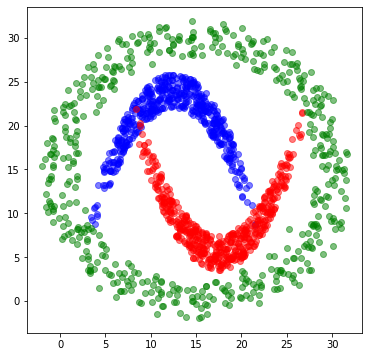Let's try to classify this data with a 5 hidden unit neural network with nonlinear logistic regression. In Python, do this by defining a new class NeuralNetworkClassifier it is easy to create a new class for using a neural network as a classifier by making a subclass*NeuralNetworkClassifier of the NeuralNetwork and make the required changes. Most of the changes will be in error_f, gradient_f, train and use methods.

In :
import neuralnetworks as nn  # from solution to A4

In :
# import sys
# sys.path = ['.'] + sys.path

In :
import neuralnetworks as nn
import mpl_toolkits.mplot3d as plt3
from matplotlib import cm

## if you edit neuralnetwork.py, force ipython to reload it by doing this.

nHidden = 
nnet = nn.NeuralNetworkClassifier(2, nHidden, 3) # 3 classes, will actually make 2-unit output layer
nnet.train(X, T, n_epochs=20000,  learning_rate=0.1)

xs = np.linspace(0, 30, 40)
x, y = np.meshgrid(xs, xs)
Xtest = np.vstack((x.flat, y.flat)).T
Ytest = nnet.use(Xtest)
predTest, probs = nnet.use(Xtest)

plt.figure(figsize=(10, 10))

plt.subplot(2, 2, 1)
plt.plot(nnet.error_trace)
plt.xlabel("Epochs")
plt.ylabel("Likelihood")

# plt.subplot(2, 2, 3)
# nnet.draw()

colors = ['red', 'green', 'blue']
plt.subplot(2, 2, 2)

for c in range(1, 4):

plt.subplot(2, 2, 4)
plt.contourf(Xtest[:, 0].reshape((40, 40)), Xtest[:, 1].reshape((40, 40)),  predTest.reshape((40, 40)),
levels = [0.5, 1.99, 2.01, 3.5],  #    levels=(0.5, 1.5, 2.5, 3.5),
colors=('red', 'green', 'blue'));

sgd: Epoch 2000 Error=0.78911
sgd: Epoch 4000 Error=0.87490
sgd: Epoch 6000 Error=0.90023
sgd: Epoch 8000 Error=0.90956
sgd: Epoch 10000 Error=0.91550
sgd: Epoch 12000 Error=0.92012
sgd: Epoch 14000 Error=0.92412
sgd: Epoch 16000 Error=0.92753
sgd: Epoch 18000 Error=0.93027
sgd: Epoch 20000 Error=0.93243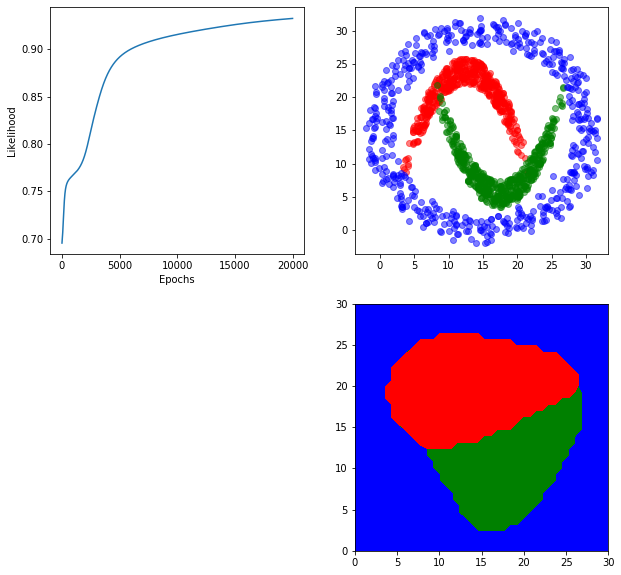In :
from matplotlib.colors import LightSource

In :
fig = plt.figure(figsize=(10, 30))
ls = LightSource(azdeg=30,  altdeg=60)
white = np.ones((x.shape,  x.shape,  3))
red = white * np.array([1, 0, 0])
green = white * np.array([0, 1, 0])
blue = white * np.array([0, 0, 1])
colors = [red,  green,  blue]

for c in range(3):
ax = fig.add_subplot(3, 1, c+1, projection='3d')
ax.view_init(azim = 180+40, elev = 60)
Z = probs[:,  c].reshape(x.shape)
ax.plot_surface(x, y, Z,
rstride=1, cstride=1, linewidth=0,  antialiased=False,
ax.set_zlabel(r"$p(C="+str(c+1)+"|x)$")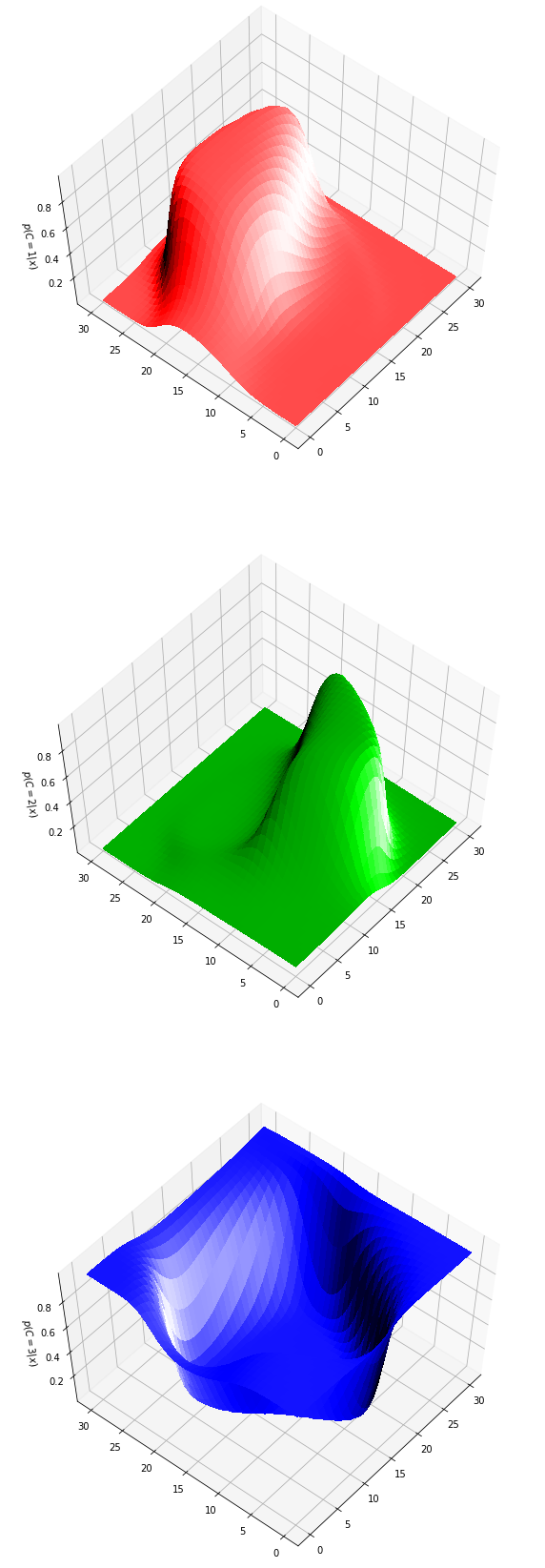How would you plot the outputs of the hidden units?

Let's repeat the experiment with classifying human activity data (accelerometers.npy), but now use our NeuralNetworkClassifier class to do nonlinear logistic regression. This time we will retrieve and load accelerometers.npy, a file containing a numpy array stored in its binary format.

In :
data = np.load('accelerometers.npy')

In :
data.shape

Out:
(225006, 4)
In :
data[0, :]

Out:
array([ 1.        , -0.87313405, -0.08552787, -0.29504612])
In :
X = data[:, 1:]
T = data[:, 0:1]
X.shape,  T.shape

Out:
((225006, 3), (225006, 1))
In :
def generate_k_fold_cross_validation_sets(X, T, n_folds, shuffle=True):

if shuffle:
# Randomly order X and T
randorder = np.arange(X.shape)
np.random.shuffle(randorder)
X = X[randorder, :]
T = T[randorder, :]

# Partition X and T into folds
n_samples = X.shape
n_per_fold = round(n_samples / n_folds)
n_last_fold = n_samples - n_per_fold * (n_folds - 1)

folds = []
start = 0
for foldi in range(n_folds-1):
folds.append( (X[start:start + n_per_fold, :], T[start:start + n_per_fold, :]) )
start += n_per_fold
folds.append( (X[start:, :], T[start:, :]) )

# Yield k(k-1) assignments of Xtrain, Train, Xvalidate, Tvalidate, Xtest, Ttest

for validation_i in range(n_folds):
for test_i in range(n_folds):
if test_i == validation_i:
continue

train_i = np.setdiff1d(range(n_folds), [validation_i, test_i])

Xvalidate, Tvalidate = folds[validation_i]
Xtest, Ttest = folds[test_i]
if len(train_i) > 1:
Xtrain = np.vstack([folds[i] for i in train_i])
Ttrain = np.vstack([folds[i] for i in train_i])
else:
Xtrain, Ttrain = folds[train_i]

yield Xtrain, Ttrain, Xvalidate, Tvalidate, Xtest, Ttest

In :
X.shape

Out:
(225006, 3)
In :
def times2():
for i in range(10):
yield i * 2

In :
list(times2())

Out:
[0, 2, 4, 6, 8, 10, 12, 14, 16, 18]
In :
z = times2()
z

Out:
<generator object times2 at 0x7f91334946d0>
In :
next(z)

Out:
0
In :
next(z)

Out:
2
In :
Xtrain,  Ttrain,  Xval, Tval, Xtest,  Ttest = next(generate_k_fold_cross_validation_sets(X,  T,  n_folds=3))

In :
Xtrain.shape, Ttrain.shape, Xval.shape, Tval.shape, Xtest.shape, Ttest.shape

Out:
((75002, 3), (75002, 1), (75002, 3), (75002, 1), (75002, 3), (75002, 1))
In :
np.unique(Ttrain,  return_counts=True)

Out:
(array([ 1.,  2.,  3.,  4.,  5.,  6.,  7.,  8.,  9., 10.]),
array([7438, 7511, 7579, 7505, 7457, 7533, 7525, 7551, 7449, 7454]))

We can control how many digits after the decimal point are printed for numpy.array values.

In :
%precision 5

Out:
'%.5f'
In :
values, counts = np.unique(Ttrain,  return_counts=True)
counts / Ttrain.shape

Out:
array([0.09917, 0.10014, 0.10105, 0.10006, 0.09942, 0.10044, 0.10033,
0.10068, 0.09932, 0.09938])
In :
values, counts = np.unique(Tval,  return_counts=True)
counts / Tval.shape

Out:
array([0.10064, 0.10025, 0.0989 , 0.10046, 0.09906, 0.09988, 0.09948,
0.09986, 0.10102, 0.10044])
In :
values, counts = np.unique(Ttest,  return_counts=True)
counts / Ttest.shape

Out:
array([0.10021, 0.0996 , 0.10004, 0.09946, 0.1015 , 0.0997 , 0.10018,
0.09945, 0.09968, 0.10017])
In :
import time

n_classes = len(np.unique(T))

start_time = time.time()

nnet = nn.NeuralNetworkClassifier(X.shape, ,  n_classes)

elapsed_time = time.time() - start_time
print(f'Training took {elapsed_time} seconds.')

plt.plot(nnet.error_trace)
plt.xlabel('Epoch')
plt.ylabel('Data Likelihood');

Adam: Epoch 10 Error=0.82802
Training took 8.751801490783691 seconds.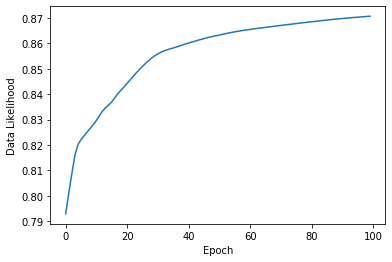For classification problems, we would like to see the percent of samples from each class that are classified corectly. It is also revealing to see to which classes the misclassified samples are assigned.

This is typically called a Confusion Matrix. This is a table of classification percents for all pairs of target classes and predicted classes. A row of the confusion matrix corresponds to a target class, and the columns are the predicted classes. So, the diagonal shows the percent of samples that are correctly classified for each target class.

In :
Classes, Probs = nnet.use(Xtrain)
table = []
for true_class in range(1, 11):
row = []
for predicted_class in range(1, 11):
row.append(100 * np.mean(Classes[Ttrain == true_class] == predicted_class))
# row.append(f'{100 * np.mean(Classes[Ttrain == true_class] == predicted_class):0.1f}')
table.append(row)
table

Out:
[[96.28932508738909,
0.13444474321054048,
1.3444474321054047,
0.013444474321054048,
2.016671148158107,
0.0,
0.0,
0.06722237160527024,
0.13444474321054048,
0.0],
[17.747303954200504,
48.422313939555316,
21.155638397017707,
2.289974703767807,
3.8210624417520966,
0.03994141925176408,
0.053255225669018774,
1.9704433497536946,
0.6257489016109705,
3.8743176674211157],
[4.182609842987201,
3.6680300831244224,
70.2599287504948,
3.351365615516559,
4.0770550204512475,
2.8103971500197917,
2.4937326824119275,
3.7735849056603774,
0.8180498746536482,
4.565246074680037],
[5.2098600932711525,
5.169886742171886,
24.290473017988006,
12.818121252498335,
12.151898734177214,
11.912058627581612,
5.902731512325117,
7.075283144570286,
5.9427048634243835,
9.526982011992006],
[10.500201153278798,
6.70510929328148,
12.189888695185731,
2.2260962853694517,
37.2938178892316,
1.3276116400697333,
1.783559072012874,
9.574896070805954,
14.57690760359394,
3.821912297170444],
[0.10619938935351123,
0.026549847338377806,
0.265498473383778,
0.23894862604540024,
0.05309969467675561,
83.71166865790521,
12.239479622992167,
0.7035709544670118,
0.1991238550378335,
2.455860878799947],
[0.0398671096345515,
0.053156146179401995,
0.770764119601329,
0.3853820598006645,
0.053156146179401995,
47.57475083056478,
43.53488372093023,
1.647840531561462,
0.132890365448505,
5.807308970099668],
[0.6621639517944643,
2.0527082505628393,
7.44272281816978,
2.3573036683882926,
8.290292676466693,
2.0659515295987285,
3.4564958283671037,
39.491458085021854,
29.86359422593034,
4.317308965699907],
[0.2953416565982011,
1.449859041482078,
5.4101221640488655,
1.664652973553497,
9.39723452812458,
1.9197207678883073,
2.4030071150489998,
34.09853671633776,
39.87112364075715,
3.4904013961605584],
[0.4024684733029246,
3.0050979339951702,
15.8304266165817,
7.04319828280118,
3.072176012878991,
20.753957606654144,
17.762275288435738,
7.620069761202039,
5.0442715320633225,
19.466058492084787]]

Of course, this is easier to read as a pandas.DataFrame.

In :
class_names = ('1-Rest', '2-Coloring', '3-Legos', '4-Wii Tennis', '5-Wii Boxing', '6-0.75m/s',
'7-1.25m/s', '8-1.75m/s', '9-2.25m/s', '10-Stairs')
class_names

Out:
('1-Rest',
'2-Coloring',
'3-Legos',
'4-Wii Tennis',
'5-Wii Boxing',
'6-0.75m/s',
'7-1.25m/s',
'8-1.75m/s',
'9-2.25m/s',
'10-Stairs')
In :
import pandas
conf_matrix = pandas.DataFrame(table, index=class_names, columns=class_names)
conf_matrix

Out:
1-Rest 2-Coloring 3-Legos 4-Wii Tennis 5-Wii Boxing 6-0.75m/s 7-1.25m/s 8-1.75m/s 9-2.25m/s 10-Stairs
1-Rest 96.289325 0.134445 1.344447 0.013444 2.016671 0.000000 0.000000 0.067222 0.134445 0.000000
2-Coloring 17.747304 48.422314 21.155638 2.289975 3.821062 0.039941 0.053255 1.970443 0.625749 3.874318
3-Legos 4.182610 3.668030 70.259929 3.351366 4.077055 2.810397 2.493733 3.773585 0.818050 4.565246
4-Wii Tennis 5.209860 5.169887 24.290473 12.818121 12.151899 11.912059 5.902732 7.075283 5.942705 9.526982
5-Wii Boxing 10.500201 6.705109 12.189889 2.226096 37.293818 1.327612 1.783559 9.574896 14.576908 3.821912
6-0.75m/s 0.106199 0.026550 0.265498 0.238949 0.053100 83.711669 12.239480 0.703571 0.199124 2.455861
7-1.25m/s 0.039867 0.053156 0.770764 0.385382 0.053156 47.574751 43.534884 1.647841 0.132890 5.807309
8-1.75m/s 0.662164 2.052708 7.442723 2.357304 8.290293 2.065952 3.456496 39.491458 29.863594 4.317309
9-2.25m/s 0.295342 1.449859 5.410122 1.664653 9.397235 1.919721 2.403007 34.098537 39.871124 3.490401
10-Stairs 0.402468 3.005098 15.830427 7.043198 3.072176 20.753958 17.762275 7.620070 5.044272 19.466058

Time for some more pandas wizardry. Help on the many options is available at this Towards Data Science post and this documentation on Pandas Styling.

In :
conf_matrix.style.format("{:.1f} %")

Out:
1-Rest 2-Coloring 3-Legos 4-Wii Tennis 5-Wii Boxing 6-0.75m/s 7-1.25m/s 8-1.75m/s 9-2.25m/s 10-Stairs
1-Rest 96.3 % 0.1 % 1.3 % 0.0 % 2.0 % 0.0 % 0.0 % 0.1 % 0.1 % 0.0 %
2-Coloring 17.7 % 48.4 % 21.2 % 2.3 % 3.8 % 0.0 % 0.1 % 2.0 % 0.6 % 3.9 %
3-Legos 4.2 % 3.7 % 70.3 % 3.4 % 4.1 % 2.8 % 2.5 % 3.8 % 0.8 % 4.6 %
4-Wii Tennis 5.2 % 5.2 % 24.3 % 12.8 % 12.2 % 11.9 % 5.9 % 7.1 % 5.9 % 9.5 %
5-Wii Boxing 10.5 % 6.7 % 12.2 % 2.2 % 37.3 % 1.3 % 1.8 % 9.6 % 14.6 % 3.8 %
6-0.75m/s 0.1 % 0.0 % 0.3 % 0.2 % 0.1 % 83.7 % 12.2 % 0.7 % 0.2 % 2.5 %
7-1.25m/s 0.0 % 0.1 % 0.8 % 0.4 % 0.1 % 47.6 % 43.5 % 1.6 % 0.1 % 5.8 %
8-1.75m/s 0.7 % 2.1 % 7.4 % 2.4 % 8.3 % 2.1 % 3.5 % 39.5 % 29.9 % 4.3 %
9-2.25m/s 0.3 % 1.4 % 5.4 % 1.7 % 9.4 % 1.9 % 2.4 % 34.1 % 39.9 % 3.5 %
10-Stairs 0.4 % 3.0 % 15.8 % 7.0 % 3.1 % 20.8 % 17.8 % 7.6 % 5.0 % 19.5 %

The above function call does not save the style in conf_matrix.

In :
conf_matrix

Out:
1-Rest 2-Coloring 3-Legos 4-Wii Tennis 5-Wii Boxing 6-0.75m/s 7-1.25m/s 8-1.75m/s 9-2.25m/s 10-Stairs
1-Rest 96.289325 0.134445 1.344447 0.013444 2.016671 0.000000 0.000000 0.067222 0.134445 0.000000
2-Coloring 17.747304 48.422314 21.155638 2.289975 3.821062 0.039941 0.053255 1.970443 0.625749 3.874318
3-Legos 4.182610 3.668030 70.259929 3.351366 4.077055 2.810397 2.493733 3.773585 0.818050 4.565246
4-Wii Tennis 5.209860 5.169887 24.290473 12.818121 12.151899 11.912059 5.902732 7.075283 5.942705 9.526982
5-Wii Boxing 10.500201 6.705109 12.189889 2.226096 37.293818 1.327612 1.783559 9.574896 14.576908 3.821912
6-0.75m/s 0.106199 0.026550 0.265498 0.238949 0.053100 83.711669 12.239480 0.703571 0.199124 2.455861
7-1.25m/s 0.039867 0.053156 0.770764 0.385382 0.053156 47.574751 43.534884 1.647841 0.132890 5.807309
8-1.75m/s 0.662164 2.052708 7.442723 2.357304 8.290293 2.065952 3.456496 39.491458 29.863594 4.317309
9-2.25m/s 0.295342 1.449859 5.410122 1.664653 9.397235 1.919721 2.403007 34.098537 39.871124 3.490401
10-Stairs 0.402468 3.005098 15.830427 7.043198 3.072176 20.753958 17.762275 7.620070 5.044272 19.466058

We can add colored backgrounds to provide quick visual comparison.

In :
conf_matrix.style.background_gradient(cmap='Blues')

Out:
1-Rest 2-Coloring 3-Legos 4-Wii Tennis 5-Wii Boxing 6-0.75m/s 7-1.25m/s 8-1.75m/s 9-2.25m/s 10-Stairs
1-Rest 96.289325 0.134445 1.344447 0.013444 2.016671 0.000000 0.000000 0.067222 0.134445 0.000000
2-Coloring 17.747304 48.422314 21.155638 2.289975 3.821062 0.039941 0.053255 1.970443 0.625749 3.874318
3-Legos 4.182610 3.668030 70.259929 3.351366 4.077055 2.810397 2.493733 3.773585 0.818050 4.565246
4-Wii Tennis 5.209860 5.169887 24.290473 12.818121 12.151899 11.912059 5.902732 7.075283 5.942705 9.526982
5-Wii Boxing 10.500201 6.705109 12.189889 2.226096 37.293818 1.327612 1.783559 9.574896 14.576908 3.821912
6-0.75m/s 0.106199 0.026550 0.265498 0.238949 0.053100 83.711669 12.239480 0.703571 0.199124 2.455861
7-1.25m/s 0.039867 0.053156 0.770764 0.385382 0.053156 47.574751 43.534884 1.647841 0.132890 5.807309
8-1.75m/s 0.662164 2.052708 7.442723 2.357304 8.290293 2.065952 3.456496 39.491458 29.863594 4.317309
9-2.25m/s 0.295342 1.449859 5.410122 1.664653 9.397235 1.919721 2.403007 34.098537 39.871124 3.490401
10-Stairs 0.402468 3.005098 15.830427 7.043198 3.072176 20.753958 17.762275 7.620070 5.044272 19.466058

And combine these two styles by applying multiple styles in object-oriented fashion.

In :
conf_matrix.style.background_gradient(cmap='Blues').format("{:.1f} %")

Out:
1-Rest 2-Coloring 3-Legos 4-Wii Tennis 5-Wii Boxing 6-0.75m/s 7-1.25m/s 8-1.75m/s 9-2.25m/s 10-Stairs
1-Rest 96.3 % 0.1 % 1.3 % 0.0 % 2.0 % 0.0 % 0.0 % 0.1 % 0.1 % 0.0 %
2-Coloring 17.7 % 48.4 % 21.2 % 2.3 % 3.8 % 0.0 % 0.1 % 2.0 % 0.6 % 3.9 %
3-Legos 4.2 % 3.7 % 70.3 % 3.4 % 4.1 % 2.8 % 2.5 % 3.8 % 0.8 % 4.6 %
4-Wii Tennis 5.2 % 5.2 % 24.3 % 12.8 % 12.2 % 11.9 % 5.9 % 7.1 % 5.9 % 9.5 %
5-Wii Boxing 10.5 % 6.7 % 12.2 % 2.2 % 37.3 % 1.3 % 1.8 % 9.6 % 14.6 % 3.8 %
6-0.75m/s 0.1 % 0.0 % 0.3 % 0.2 % 0.1 % 83.7 % 12.2 % 0.7 % 0.2 % 2.5 %
7-1.25m/s 0.0 % 0.1 % 0.8 % 0.4 % 0.1 % 47.6 % 43.5 % 1.6 % 0.1 % 5.8 %
8-1.75m/s 0.7 % 2.1 % 7.4 % 2.4 % 8.3 % 2.1 % 3.5 % 39.5 % 29.9 % 4.3 %
9-2.25m/s 0.3 % 1.4 % 5.4 % 1.7 % 9.4 % 1.9 % 2.4 % 34.1 % 39.9 % 3.5 %
10-Stairs 0.4 % 3.0 % 15.8 % 7.0 % 3.1 % 20.8 % 17.8 % 7.6 % 5.0 % 19.5 %

Okay. Let's try a bigger network. Maybe we can increase some of those accuracies along the diagonal.

In :
start_time = time.time()

nnet = nn.NeuralNetworkClassifier(X.shape, [100, 50],  n_classes)

elapsed_time = time.time() - start_time
print(f'Training took {elapsed_time} seconds.')

plt.plot(nnet.error_trace)
plt.xlabel('Epoch')
plt.ylabel('Data Likelihood');

Adam: Epoch 100 Error=0.88669
Training took 553.6346580982208 seconds.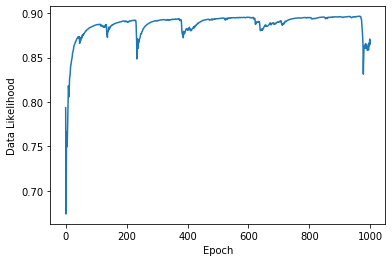In :
Classes, Probs = nnet.use(Xtrain)
table = []
for true_class in range(1, 11):
row = []
for predicted_class in range(1, 11):
row.append(100 * np.mean(Classes[Ttrain == true_class] == predicted_class))
# row.append(f'{100 * np.mean(Classes[Ttrain == true_class] == predicted_class):0.1f}')
table.append(row)

print(f'Train percent correct {np.mean(Classes == Ttrain) * 100:.2f}')

conf_matrix = pandas.DataFrame(table, index=class_names, columns=class_names)

Train percent correct 46.55

Out:
1-Rest 2-Coloring 3-Legos 4-Wii Tennis 5-Wii Boxing 6-0.75m/s 7-1.25m/s 8-1.75m/s 9-2.25m/s 10-Stairs
1-Rest 54.8 % 42.9 % 0.1 % 1.8 % 0.0 % 0.0 % 0.0 % 0.0 % 0.3 % 0.0 %
2-Coloring 9.0 % 73.6 % 5.0 % 4.0 % 1.1 % 0.0 % 0.1 % 0.0 % 5.6 % 1.5 %
3-Legos 10.2 % 3.9 % 53.4 % 15.9 % 0.4 % 2.2 % 1.0 % 0.7 % 2.8 % 9.5 %
4-Wii Tennis 7.0 % 5.4 % 14.8 % 24.3 % 2.8 % 7.9 % 2.6 % 2.6 % 9.4 % 23.3 %
5-Wii Boxing 12.3 % 6.3 % 6.6 % 18.5 % 25.4 % 0.9 % 0.6 % 6.1 % 16.5 % 6.8 %
6-0.75m/s 0.1 % 0.1 % 0.2 % 0.5 % 0.0 % 77.8 % 7.0 % 0.2 % 0.8 % 13.2 %
7-1.25m/s 0.0 % 0.2 % 0.6 % 1.3 % 0.1 % 41.2 % 35.7 % 0.6 % 1.0 % 19.1 %
8-1.75m/s 1.4 % 0.6 % 4.2 % 9.1 % 4.3 % 1.6 % 2.5 % 22.8 % 43.8 % 9.8 %
9-2.25m/s 0.7 % 0.6 % 3.6 % 7.3 % 6.1 % 1.7 % 1.5 % 18.7 % 53.6 % 6.1 %
10-Stairs 0.4 % 3.4 % 9.1 % 7.7 % 2.1 % 13.8 % 9.9 % 3.2 % 6.5 % 43.9 %

Now let's check the validationa and test sets.

In :
Classes, Probs = nnet.use(Xval)
table = []
for true_class in range(1, 11):
row = []
for predicted_class in range(1, 11):
row.append(100 * np.mean(Classes[Tval == true_class] == predicted_class))
# row.append(f'{100 * np.mean(Classes[Ttrain == true_class] == predicted_class):0.1f}')
table.append(row)

print(f'Val percent correct {np.mean(Classes == Tval) * 100:.2f}')

conf_matrix = pandas.DataFrame(table, index=class_names, columns=class_names)

Val percent correct 46.79

Out:
1-Rest 2-Coloring 3-Legos 4-Wii Tennis 5-Wii Boxing 6-0.75m/s 7-1.25m/s 8-1.75m/s 9-2.25m/s 10-Stairs
1-Rest 55.4 % 42.3 % 0.1 % 1.9 % 0.0 % 0.0 % 0.0 % 0.0 % 0.3 % 0.0 %
2-Coloring 9.6 % 73.6 % 5.2 % 3.5 % 1.4 % 0.0 % 0.1 % 0.0 % 4.9 % 1.7 %
3-Legos 10.8 % 4.6 % 52.8 % 15.5 % 0.5 % 2.0 % 1.0 % 0.9 % 2.7 % 9.4 %
4-Wii Tennis 6.3 % 6.1 % 14.3 % 24.4 % 3.1 % 8.2 % 2.8 % 2.4 % 8.8 % 23.6 %
5-Wii Boxing 12.0 % 6.7 % 6.3 % 18.4 % 26.0 % 0.8 % 1.0 % 6.3 % 16.4 % 6.0 %
6-0.75m/s 0.1 % 0.1 % 0.3 % 0.5 % 0.1 % 78.3 % 7.5 % 0.1 % 0.7 % 12.3 %
7-1.25m/s 0.0 % 0.1 % 0.7 % 1.2 % 0.1 % 40.9 % 36.2 % 0.5 % 0.9 % 19.4 %
8-1.75m/s 1.3 % 0.9 % 4.3 % 8.8 % 4.3 % 1.6 % 2.4 % 23.2 % 43.3 % 9.9 %
9-2.25m/s 0.8 % 0.5 % 3.3 % 7.0 % 6.3 % 1.6 % 1.4 % 19.1 % 54.2 % 5.8 %
10-Stairs 0.3 % 3.3 % 9.2 % 7.3 % 2.2 % 14.2 % 10.4 % 3.1 % 6.2 % 43.7 %
In :
Classes, Probs = nnet.use(Xtest)
table = []
for true_class in range(1, 11):
row = []
for predicted_class in range(1, 11):
row.append(100 * np.mean(Classes[Ttest == true_class] == predicted_class))
# row.append(f'{100 * np.mean(Classes[Ttrain == true_class] == predicted_class):0.1f}')
table.append(row)

print(f'Test percent correct {np.mean(Classes == Ttest) * 100:.2f}')

conf_matrix = pandas.DataFrame(table, index=class_names, columns=class_names)

Test percent correct 46.27

Out:
1-Rest 2-Coloring 3-Legos 4-Wii Tennis 5-Wii Boxing 6-0.75m/s 7-1.25m/s 8-1.75m/s 9-2.25m/s 10-Stairs
1-Rest 55.1 % 42.4 % 0.1 % 2.0 % 0.0 % 0.0 % 0.0 % 0.0 % 0.3 % 0.0 %
2-Coloring 9.1 % 73.8 % 5.0 % 3.5 % 1.3 % 0.0 % 0.0 % 0.0 % 5.2 % 2.1 %
3-Legos 10.0 % 4.2 % 52.2 % 17.0 % 0.3 % 2.1 % 1.1 % 0.8 % 2.7 % 9.6 %
4-Wii Tennis 6.8 % 5.9 % 15.0 % 23.9 % 2.9 % 9.3 % 2.7 % 2.6 % 8.7 % 22.3 %
5-Wii Boxing 11.4 % 7.1 % 6.3 % 18.3 % 25.8 % 1.1 % 0.6 % 6.4 % 16.6 % 6.4 %
6-0.75m/s 0.1 % 0.1 % 0.3 % 0.7 % 0.1 % 77.5 % 7.6 % 0.3 % 0.7 % 12.6 %
7-1.25m/s 0.0 % 0.3 % 0.5 % 1.4 % 0.1 % 40.5 % 35.9 % 0.4 % 1.1 % 19.7 %
8-1.75m/s 1.3 % 0.6 % 4.5 % 8.7 % 4.2 % 1.6 % 2.3 % 23.0 % 44.2 % 9.6 %
9-2.25m/s 0.9 % 0.6 % 3.0 % 7.1 % 6.7 % 1.4 % 1.5 % 19.6 % 53.0 % 6.3 %
10-Stairs 0.6 % 3.6 % 9.1 % 8.4 % 2.2 % 14.1 % 9.8 % 3.0 % 6.4 % 42.8 %

What about a different data representation, maybe one involving frequencies to represent movement?

One such representation is based on the continuous wavelet transform, or cwt. Here is some code that applies a cwt to our accelerometer data.

In :
import scipy.signal as sig

def cwt(eeg, Fs, freqs, width, channelNames=None, graphics=False):
if freqs.min() == 0:
print('cwt: Frequencies must be greater than 0.')
return None, None
nChannels, nSamples = eeg.shape
if not channelNames and graphics:
channelNames = [f'Channel {i:2d}' for i in range(nChannels)]

nFreqs = len(freqs)
tfrep = np.zeros((nChannels,  nFreqs, nSamples))
tfrepPhase = np.zeros((nChannels, nFreqs, nSamples))

for ch in range(nChannels):
print('channel', ch, ' freq ', end='')
for freqi in range(nFreqs):
print(freqs[freqi], ' ', end='')
mag, phase = energyvec(freqs[freqi], eeg[ch, :], Fs, width)
tfrepPhase[ch, freqi, :] = phase
tfrep[ch, freqi, :] = mag
print()

return tfrep, tfrepPhase

def morletLength(Fs, f, width):
''' len = morletLength(Fs, f, width) '''
dt = 1.0/Fs
sf = f/width
st = 1.0/(2*np.pi*sf)
return int((3.5*st - -3.5*st)/dt)

def energyvec(f, s, Fs, width):
'''
function [y, phase] <- energyvec(f, s, Fs, width)
function y <- energyvec(f, s, Fs, width)

Return a vector containing the energy as a
function of time for frequency f. The energy
is calculated using Morlet''s wavelets.
s : signal
Fs: sampling frequency
width : width of Morlet wavelet (><- 5 suggested).
'''

dt = 1.0 / Fs
sf = f / width
st = 1.0 / (2 * np.pi * sf)

t = np.arange(-3.5 * st, 3.5 * st, step=dt)
m = morlet(f, t, width)
# yconv = np.convolve(s, m, mode="same")
yconv = sig.fftconvolve(s, m, mode='same')

lengthMorlet = len(m)
firsthalf = int(lengthMorlet / 2.0 + 0.5)
secondhalf = lengthMorlet - firsthalf

yconvNoBoundary = yconv
y = np.abs(yconvNoBoundary)**2
phase = np.angle(yconvNoBoundary, deg=True)
return y, phase

######################################################################

def morlet(f, t, width):
'''
function y <- morlet(f, t, width)
Morlet''s wavelet for frequency f and time t.
The wavelet will be normalized so the total energy is 1.
width defines the width of the wavelet.
A value ><- 5 is suggested.

Ref: Tallon-Baudry et al., J. Neurosci. 15, 722-734 (1997), page 724

Ole Jensen, August 1998
'''
sf = f / width
st = 1.0 / (2 * np.pi * sf)
A = 1.0 / np.sqrt(st * np.sqrt(2 * np.pi))
y = A * np.exp(-t ** 2 / (2 * st ** 2)) * np.exp(1j * 2 * np.pi * f * t)
return y

In :
width = 75
maxFreq = 20
freqs = np.arange(0.5, maxFreq, 0.5) # makes same freqs used in stft above
start_time = time.time()
tfrep, tfrepPhase = cwt(data[:, 1:].T,  75,  freqs,  width)
elapsed = time.time() - start_time
print(f'CWT time: {elapsed} seconds')

channel 0  freq 0.5  1.0  1.5  2.0  2.5  3.0  3.5  4.0  4.5  5.0  5.5  6.0  6.5  7.0  7.5  8.0  8.5  9.0  9.5  10.0  10.5  11.0  11.5  12.0  12.5  13.0  13.5  14.0  14.5  15.0  15.5  16.0  16.5  17.0  17.5  18.0  18.5  19.0  19.5
channel 1  freq 0.5  1.0  1.5  2.0  2.5  3.0  3.5  4.0  4.5  5.0  5.5  6.0  6.5  7.0  7.5  8.0  8.5  9.0  9.5  10.0  10.5  11.0  11.5  12.0  12.5  13.0  13.5  14.0  14.5  15.0  15.5  16.0  16.5  17.0  17.5  18.0  18.5  19.0  19.5
channel 2  freq 0.5  1.0  1.5  2.0  2.5  3.0  3.5  4.0  4.5  5.0  5.5  6.0  6.5  7.0  7.5  8.0  8.5  9.0  9.5  10.0  10.5  11.0  11.5  12.0  12.5  13.0  13.5  14.0  14.5  15.0  15.5  16.0  16.5  17.0  17.5  18.0  18.5  19.0  19.5
CWT time: 4.003356218338013 seconds

In :
plt.figure(figsize=(15, 15))
plt.subplot(5, 1, 1)
plt.plot(data[:, 1:])
plt.axis('tight')

plt.subplot(5, 1, 2)
plt.plot(data[:, 0])
plt.text(5000, 8, '1-Rest, 2-Coloring, 3-Legos, 4-Wii Tennis, 5-Boxing, 6-0.75, 7-1.25 m/s, 8-1.75, 9-2.25 m/s, 10-stairs')
plt.axis('tight')

nSensors = data.shape - 1
for i in range(nSensors):
plt.subplot(5, 1, i+3)
# Make a colored image of the logarithm of the CWT.
plt.imshow(np.log(tfrep[i, :, :]),
interpolation='nearest', origin='lower',
cmap=plt.cm.jet) #plt.cm.Reds)
plt.xlabel('Seconds')
plt.ylabel('Frequency in ' + ('$x$', '$y$', '$z$')[i])
tickstep = round(len(freqs) / 5)
plt.yticks(np.arange(len(freqs))[::tickstep],
[str(i) for i in freqs[::tickstep]])
plt.axis('auto')
plt.axis('tight')